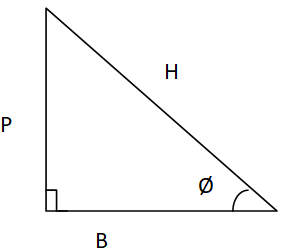Courses
Courses for Kids
Free study material
Free LIVE classes
MoreLIVE
Join Vedantu’s FREE Mastercalss

# If given a trigonometric equation$\sqrt 3 \tan \theta = 3\sin \theta$, find the value of ${\sin ^2}\theta - {\cos ^2}\theta$Verified
366.3k+ views
Hint: - Use the trigonometric identities and Pythagoras theorem.

Given:$\sqrt 3 \tan \theta = 3\sin \theta$
$\Rightarrow \tan \theta = \dfrac{3}{{\sqrt 3 }}\sin \theta \\ \Rightarrow \tan \theta = \sqrt 3 \sin \theta \\ \Rightarrow \dfrac{{\tan \theta }}{{\sin \theta }} = \sqrt 3 \\ \Rightarrow \cos \theta = \dfrac{1}{{\sqrt 3 }} \\ \Rightarrow \cos \theta = \dfrac{{Adjacent{\text{ }}side}}{{Hypotenuse}} = \dfrac{B}{H} = \dfrac{1}{{\sqrt 3 }} \\$From the above figure for the Right angled triangle by using Pythagoras Theorem,
${H^2} = {P^2} + {B^2} \\ {\left( {\sqrt 3 } \right)^2} = {P^2} + {1^2} \\ {P^2} = 3 - 1 \\ {P^2} = 2 \\ P = \sqrt 2 \\$
Now, ${\sin ^2}\theta - {\cos ^2}\theta = {\left( {\dfrac{P}{H}} \right)^2} - {\left( {\dfrac{B}{H}} \right)^2}$
$= {\left( {\dfrac{{\sqrt 2 }}{{\sqrt 3 }}} \right)^2} - {\left( {\dfrac{1}{{\sqrt 3 }}} \right)^2} \\ = \dfrac{2}{3} - \dfrac{1}{3} \\ = \dfrac{1}{3} \\$
Note: The above question can be solved by using trigonometric identities, but here it is done by visualizing the terms in the form of sides of the right angled triangle, thus making the problem easier to solve.
Last updated date: 01st Oct 2023
Total views: 366.3k
Views today: 8.66k